## Pages

Showing posts with label formula. Show all posts
Showing posts with label formula. Show all posts

If a quadratic equation factors then certainly you should solve it by factoring. The problem is that not all quadratic equations factor. When this is the case we could use the quadratic formula to find the solutions. (We will limit our introduction to equations where b^2 - 4ac >= 0 .)Most textbooks provide a nice proof of the quadratic formula. The proof involves solving the general quadratic by completing the square. This study guide will focus on using the formula to solve problems.

Solve using the quadratic formula:Step 1: Identify the coefficients a, b, and c.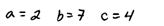Step 2: Write down the formula (you should memorize it).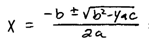Step 3: Substitute in the appropriate values and then evaluate.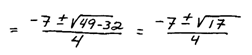Solve using the quadratic formula.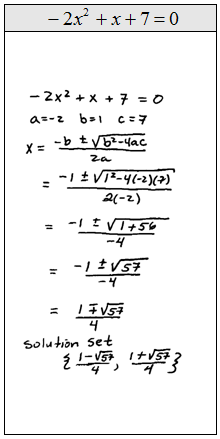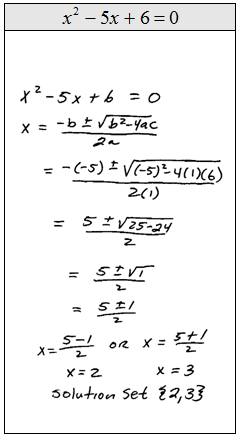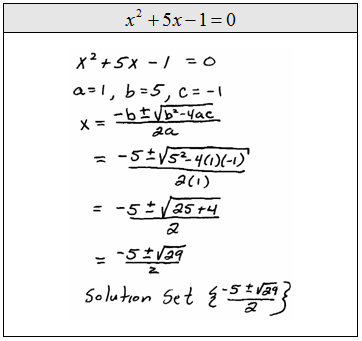Video Examples on YouTube:### Distance and Midpoint Formulas

In this section we will review the distance and midpoint formulas.
Notice that the distance formula results in a real number and that the midpoint formula results in an ordered pair.

[ Interactive: Distance and Midpoint ]

Given two points find the distance and midpoint between them.

Video Lessons:
Use the distance and/or midpoint formulas to solve the following.

Example: If the diameter of a circle is defined by the two points (-3, 4) and (7, 4), find the center and radius of the circle. (Hint: diameter = 2r)
Example: Find the area of a circle given center (-3, 3) and point (3, 3) on the circle.
Video Examples on YouTube:
YouTube Example: Find the coordinates of an endpoint given the other endpoint and the midpoint.
---